# Preface

This tutorial was made solely for the purpose of education and it was designed for students taking Applied Math 0340. It is primarily for students who have some experience using Mathematica. If you have never used Mathematica before and would like to learn more of the basics for this computer algebra system, it is strongly recommended to look at the APMA 0330 tutorial. As a friendly reminder, don't forget to clear variables in use and/or the kernel. The Mathematica commands in this tutorial are all written in bold black font, while Mathematica output is in regular fonts.

Finally, you can copy and paste all commands into your Mathematica notebook, change the parameters, and run them because the tutorial is under the terms of the GNU General Public License (GPL). You, as the user, are free to use the scripts for your needs to learn the Mathematica program, and have the right to distribute this tutorial and refer to this tutorial as long as this tutorial is accredited appropriately. The tutorial accompanies the textbook Applied Differential Equations. The Primary Course by Vladimir Dobrushkin, CRC Press, 2015; http://www.crcpress.com/product/isbn/9781439851043

Introduction to Linear Algebra with Mathematica

# Forced anharmonic motion

We consider the following unharmonic oscillator:
$x'' + x(t) - \frac{1}{6}\, x^3 =0.3\,\cos \left( 0.5\,t \right) , \qquad x(0) = a, \quad x' (0) = b .$
Not for arbitrary values of initial parameters (𝑎,b) the given initial value problem has a bounded solution. So we check with Mathematica.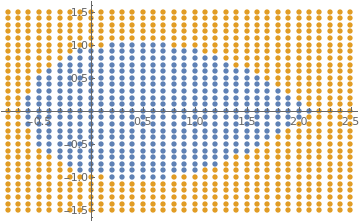pfun = ParametricNDSolveValue[{x''[t] == -x[t] + x[t]^3/6 + 0.3*Cos[0.5*t], x == a, x' == b}, x, {t, 0, 100}, {a, b}]; allPars = Flatten[Chop[ Table[{a, b}, {a, -0.8, 2.5, 0.1}, {b, -1.5, 1.5, 0.1}]], {1, 2}];; validPars = {}; invalidPars = {}; Table[If[Apply[pfun, par]["Domain"] === {{0., 100.}}, AppendTo[validPars, par], AppendTo[invalidPars, par]], {par, allPars}]; ListPlot[{validPars, invalidPars}, PlotLegends -> {"Valid Parameters", "Invalid Parameters"}, PlotStyle -> {Directive[PointSize[0.015]]}] Region of bounded solutions Mathematica code

There is another approach: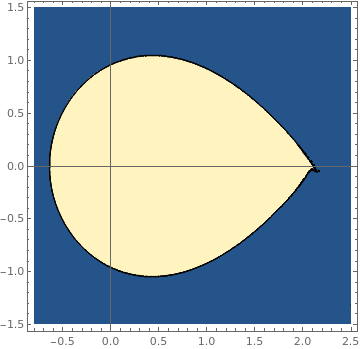fun1[a_?NumericQ, b_?NumericQ] := Module[ {res},; (* determine if domain is valid or invalid and return 1 or 0 respectively *); res = Quiet[pfun[a, b]];; Boole[res["Domain"] === {{0., 100.}}]; ];; ContourPlot[fun1[a, b], {a, -0.8, 2.5}, {b, -1.5, 1.5}, PlotPoints -> 50, MaxRecursion -> 3, Axes -> True, AxesOrigin -> {0, 0}] Region of bounded solutions Mathematica code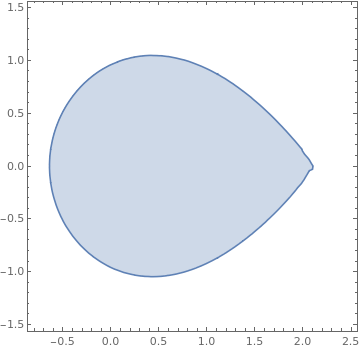We make a region using the boolean function defined earlier regionplot = RegionPlot[fun[a, b] >= 1, {a, -0.6, 2}, {b, -1, 1}] Region of bounded solutions Mathematica code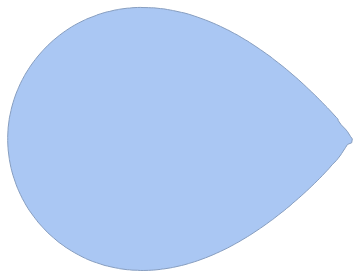Create a boundary mesh from the region mesh = BoundaryDiscretizeGraphics[regionplot] Boundary of the domain Mathematica code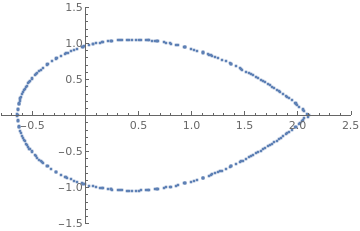Now we generate the set of coordinates; however, they are not ordered. As you increase n using the slider, the curve fills up in random spots Manipulate[ ListPlot[coord[[1 ;; n]], PlotRange -> {{-0.8, 2.5}, {-1.5, 1.5}}], {{n, 50}, 1, Length[coord], 1} ] Boundary of the region Mathematica code

Next we sort the boundary coordinates. MeshCells[] will give the lines that connect different points. Note that the arguments for Line[] are the coordinate indices (not the coordinate positions)
meshLines = MeshCells[mesh, 1]; meshLines // Short
{Line[{1,2}], Line[{2,42}], Line[{42.40}], >>272<<, Line[{122,123}], Line[{123,1}]}
Get the order of points
orderOfPoints = (Apply[Sequence, #] & /@ meshLines)[[All, 1]]; orderOfPoints // Short
{1, 2, 42, 41, 221, 92,34, 35, 36, 37, >>252<<, 27, 275, 214, 215, 229, 230, 217, 218, 157, 122, 123}
Sort the coordinates we obtained earlier
sortedcoord = coord[[orderOfPoints]];
The points are then further ordered so we start at the right end of the shape (this is optional)
While[sortedcoord[[1, 1]] != Max[sortedcoord[[All, 1]]], sortedcoord = RotateLeft[sortedcoord, 1]; ]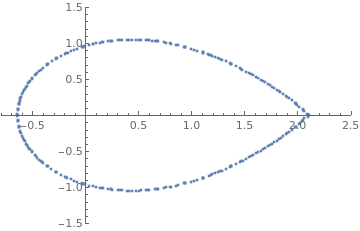Use the slider below to see that the points are now ordered Manipulate[ ListPlot[sortedcoord[[1 ;; n]], PlotRange -> {{-0.8, 2.5}, {-1.5, 1.5}}], {{n, 50}, 1, Length[sortedcoord], 1} ] The boundary of the domain, Mathematica code

Parametrizing the x- and y- coordinates separately in terms of pairs. Find the distance between each successive pair of points, and then the distances are added up cumulatively using Accumulate[] (this will be the parameter for the x- and y- coordinates).
dist = Accumulate[Prepend[Norm /@ Differences[sortedcoord], 0.]];
Building the list of points {{p1, x1}, {p2, x2}.. {pn, xn}} and {{p1, y1}, {p2, y2}.. {pn, yn}} and fitting with an interpolation function.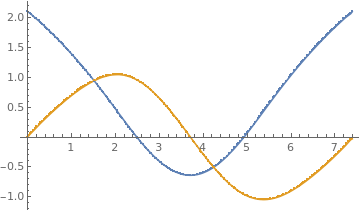{px, py} = Transpose[{dist, #}] & /@ Transpose[sortedcoord]; {funca, funcb} = Interpolation[#, InterpolationOrder -> 1] & /@ {px, py}; Show[ Plot[{funca[p], funcb[p]}, {p, 0, dist[[-1]]}], ListPlot[{px, py}, PlotLegends -> {"\!$$\*SubscriptBox[\(p$$, \ $$i$$]\),\!$$\*SubscriptBox[\(x$$, $$i$$]\)", "\!$$\*SubscriptBox[\(p$$, $$i$$]\),\!$$\*SubscriptBox[\(y$$, $$i\$$]\)"}] ] Plot of the boundary coordinates         separately. Mathematica code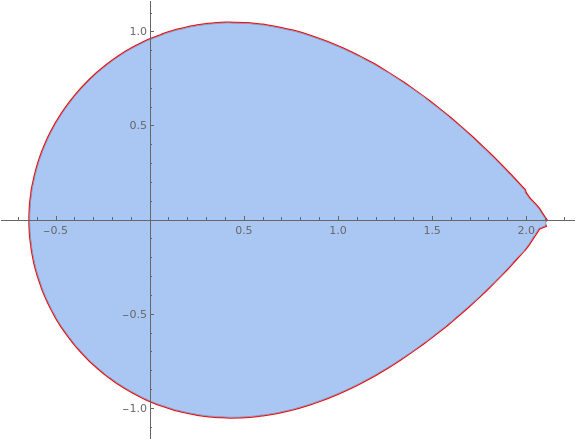See the parametric plot of the interpolation function and compare to the region Show[ ParametricPlot[{funca[p], funcb[p]}, {p, 0, dist[[-1]]}, PlotStyle -> {Thickness[0.005], Red}, ImageSize -> Large], mesh ] The boundary of the domain, Mathematica code

(* F = 1 *)
s = NDSolve[{x''[t]== -x[t] - x[t]^3 + 1*Cos[t], x==1, x'==0},x,{t,0,100}] ParametricPlot[Evaluate[{x[t],x'[t]}/.s],{t,0,100}]

(* F = 1.5 *)
s2 = NDSolve[{x''[t]== -x[t] - x[t]^3 + 1.5*Cos[t], x==1, x'==0},x,{t,0,100}] ParametricPlot[Evaluate[{x[t],x'[t]}/.s2],{t,0,100}]

(* F = 2.1 *)
s3 = NDSolve[{x''[t]== -x[t] - x[t]^3 + 2.1*Cos[t], x==1, x'==0},x,{t,0,100}] ParametricPlot[Evaluate[{x[t],x'[t]}/.s3],{t,0,100}]

(* F = 3 *)
s4 = NDSolve[{x''[t]== -x[t] - x[t]^3 + 3*Cos[t], x==1, x'==0},x,{t,0,100}] ParametricPlot[Evaluate[{x[t],x'[t]}/.s4],{t,0,100}]

(* omega = 2 *)
(* F = 1 *)
w = NDSolve[{x''[t]== -x[t] - x[t]^3 + 1*Cos[2*t], x==1, x'==0},x,{t,0,100}] ParametricPlot[Evaluate[{x[t],x'[t]}/.w],{t,0,100}]

(* F = 0.5 *)
w2 = NDSolve[{x''[t]== -x[t] - x[t]^3 + 0.5*Cos[2*t], x==1, x'==0},x,{t,0,100}] ParametricPlot[Evaluate[{x[t],x'[t]}/.w2],{t,0,100}]

(* F = 4.3 *)
w3 = NDSolve[{x''[t]== -x[t] - x[t]^3 + 4.3*Cos[2*t], x==1, x'==0},x,{t,0,100}] ParametricPlot[Evaluate[{x[t],x'[t]}/.w3],{t,0,100}]

(* F = 5 *)
w4 = NDSolve[{x''[t]== -x[t] - x[t]^3 + 5*Cos[2*t], x==1, x'==0},x,{t,0,100}] ParametricPlot[Evaluate[{x[t],x'[t]}/.w4],{t,0,100}]